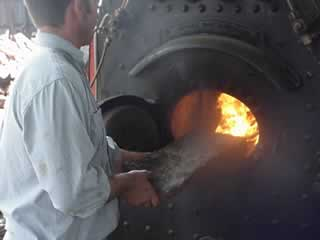Thermochemistry IndexActivation energy - activation energy (Flash animation) Biofuels Calorimeter -calibrations Chemical reactions Energy profiles Endothermic reactions -demonstration of endothermic reaction Enthalpy calculations with related equations - enthalpy calculations using CuSO4 Exothermic reactions Hess' Law Latent heat Phase change diagrams Photosynthesis Power and energy transformations -electrical energy production Respiration Specific heat capacity ---Exercises ---Simple exercises Thermochemistry (energy released in chemical reactions) Thermochemical equations and exercises -past exam question